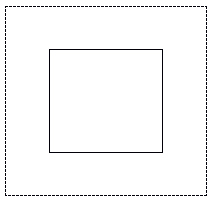﻿ LocalHistogramEqualizeCommand Class | Leadtools.ImageProcessing.Color | Raster, Medical, Document Help
←Select platform
In This Topic ▼

# LocalHistogramEqualizeCommand Class

Summary

Linearizes the number of pixels locally in an image, based on a specific color space. This can be used to bring out the detail in dark areas of an image, and smooth the edges between blocks. This class is available in the Medical Toolkits.

Syntax
C#
VB
Java
Objective-C
C++
``public class LocalHistogramEqualizeCommand : RasterCommand ``
````Public Class LocalHistogramEqualizeCommand  `
`   Inherits RasterCommand ````
``@interface LTLocalHistogramEqualizeCommand : LTRasterCommand ``
``public class LocalHistogramEqualizeCommand extends RasterCommand ``
``public ref class LocalHistogramEqualizeCommand : public RasterCommand   ``

Remarks
• This class applies the histogram equalizer locally on the image. The image is divided into local rectangles of dimension (Width, Height). The number of pixels inside each local rectangle are linearized according the histogram calculated for an extended rectangle around each local rectangle. The extended rectangles are of dimension: ((Width+2 * WidthExtension) , ( Height + 2 * HeightExtension)). For an example, see the following drawing:A rectangle and its associated extension, where the histogram is calculated.

• Use this class to bring out details in an image that has portions that are too dark or too bright. An example of this is a picture taken with a flashlight in a dark room. In such a picture, the subject receives good light while the background is very dark. The application of the local histogram brings out details, but creates a blocky artifact. Use Smooth to reduce the blockiness of the result.

• This class supports 12 and 16-bit grayscale and 48 and 64-bit color images. Support for 12 and 16-bit grayscale and 48 and 64-bit color images is available only in the Document/Medical toolkits.
• This command does not support 32-bit grayscale images.
• This command supports signed/unsigned data images.

Example

Run the LocalHistogramEqualizeCommand on an image.

C#
VB
````using Leadtools; `
`using Leadtools.Codecs; `
`using Leadtools.ImageProcessing.Color; `
` `
`public void LocalHistogramEqualizeCommandExample() `
`{ `
`   // Load an image `
`   RasterCodecs codecs = new RasterCodecs(); `
`   codecs.ThrowExceptionsOnInvalidImages = true; `
` `
`   RasterImage image = codecs.Load(Path.Combine(LEAD_VARS.ImagesDir, "IMAGE1.CMP")); `
` `
`   // Prepare the command `
`   LocalHistogramEqualizeCommand command = new LocalHistogramEqualizeCommand(); `
`   command.Width = 15; `
`   command.Height = 15; `
`   command.WidthExtension = 100; `
`   command.HeightExtension = 100; `
`   command.Smooth = 0; `
`   command.Type = HistogramEqualizeType.Yuv; `
`   // Local Histogram equalize the image. `
`   command.Run(image); `
`   codecs.Save(image, Path.Combine(LEAD_VARS.ImagesDir, "Result.jpg"), RasterImageFormat.Jpeg, 24); `
` `
`} `
` `
`static class LEAD_VARS `
`{ `
`   public const string ImagesDir = @"C:\Users\Public\Documents\LEADTOOLS Images"; `
`} ````
````Imports Leadtools `
`Imports Leadtools.Codecs `
`Imports Leadtools.ImageProcessing.Color `
` `
`Public Sub LocalHistogramEqualizeCommandExample() `
`   Dim codecs As New RasterCodecs() `
`   codecs.ThrowExceptionsOnInvalidImages = True `
` `
`   Dim leadImage As RasterImage = codecs.Load(Path.Combine(LEAD_VARS.ImagesDir, "IMAGE1.CMP")) `
` `
`   ' Prepare the command `
`   Dim command As LocalHistogramEqualizeCommand = New LocalHistogramEqualizeCommand `
`   command.Width = 15 `
`   command.Height = 15 `
`   command.WidthExtension = 100 `
`   command.HeightExtension = 100 `
`   command.Smooth = 0 `
`   command.Type = HistogramEqualizeType.Yuv `
`   ' Local Histogram equalize the image. `
`   command.Run(leadImage) `
`   codecs.Save(leadImage, Path.Combine(LEAD_VARS.ImagesDir, "Result.jpg"), RasterImageFormat.Jpeg, 24) `
` `
`End Sub `
` `
`Public NotInheritable Class LEAD_VARS `
`   Public Const ImagesDir As String = "C:\Users\Public\Documents\LEADTOOLS Images" `
`End Class ````
````c#[Silverlight C# Example] `
`using Leadtools; `
`using Leadtools.Codecs; `
`using Leadtools.ImageProcessing.Color; `
`using Leadtools.Examples; `
` `
`public void LocalHistogramEqualizeCommandExample(RasterImage image, Stream outStream) `
`{ `
`   // Prepare the command `
`   LocalHistogramEqualizeCommand command = new LocalHistogramEqualizeCommand(); `
`   command.Width = 15; `
`   command.Height = 15; `
`   command.WidthExtension = 100; `
`   command.HeightExtension = 100; `
`   command.Smooth = 0; `
`   command.Type = HistogramEqualizeType.Yuv; `
`   // Local Histogram equalize the image. `
`   command.Run(image); `
` `
`   // Save result image `
`   RasterCodecs codecs = new RasterCodecs(); `
`   codecs.Save(image, outStream, RasterImageFormat.Jpeg, 24); `
`   image.Dispose(); `
`} `
`vb[Silverlight VB Example] `
`Imports Leadtools `
`Imports Leadtools.Codecs `
`Imports Leadtools.ImageProcessing.Color `
` `
`Public Sub LocalHistogramEqualizeCommandExample(ByVal image As RasterImage, ByVal outStream As Stream) `
`   ' Prepare the command `
`   Dim command As LocalHistogramEqualizeCommand = New LocalHistogramEqualizeCommand() `
`   command.Width = 15 `
`   command.Height = 15 `
`   command.WidthExtension = 100 `
`   command.HeightExtension = 100 `
`   command.Smooth = 0 `
`   command.Type = HistogramEqualizeType.Yuv `
`   ' Local Histogram equalize the image. `
`   command.Run(image) `
` `
`   ' Save result image `
`   Dim codecs As RasterCodecs = New RasterCodecs() `
`   codecs.Save(image, outStream, RasterImageFormat.Jpeg, 24) `
`   image.Dispose() `
`End Sub ````

Requirements

Help Version 20.0.2019.9.19